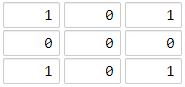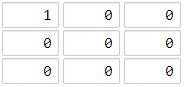## Algorithm

Problem Name: 1162. As Far from Land as Possible

Given an `n x n` `grid` containing only values `0` and `1`, where `0` represents water and `1` represents land, find a water cell such that its distance to the nearest land cell is maximized, and return the distance. If no land or water exists in the grid, return `-1`.

The distance used in this problem is the Manhattan distance: the distance between two cells `(x0, y0)` and `(x1, y1)` is `|x0 - x1| + |y0 - y1|`.

Example 1:```Input: grid = [[1,0,1],[0,0,0],[1,0,1]]
Output: 2
Explanation: The cell (1, 1) is as far as possible from all the land with distance 2.
```

Example 2:```Input: grid = [[1,0,0],[0,0,0],[0,0,0]]
Output: 4
Explanation: The cell (2, 2) is as far as possible from all the land with distance 4.
```

Constraints:

• `n == grid.length`
• `n == grid[i].length`
• `1 <= n <= 100`
• `grid[i][j]` is `0` or `1`

## Code Examples

### #1 Code Example with Java Programming

```Code - Java Programming```

``````
class Solution {
private final int[][] DIRS = {{1, 0}, {-1, 0}, {0, -1}, {0, 1}};

public int maxDistance(int[][] grid) {
int numRows = grid.length;
int numCols = grid.length;
for (int i = 0; i < numRows; i++) {
for (int j = 0; j < numCols; j++) {
if (grid[i][j] == 1) {
}
}
}
if (queue.isEmpty() || queue.size() == numRows * numCols) {
return -1;
}
int distance = 0;
while (!queue.isEmpty()) {
int size = queue.size();
while (size-- > 0) {
int[] removed = queue.remove();
for (int[] dir : DIRS) {
int x = removed + dir;
int y = removed + dir;
if (x < 0 || y < 0 || x >= numRows || y >= numCols || grid[x][y] == 1) {
continue;
}
grid[x][y] = 1;
}
}
distance++;
}
return distance - 1;
}
}
``````
Copy The Code &

Input

cmd
grid = [[1,0,1],[0,0,0],[1,0,1]]

Output

cmd
2

### #2 Code Example with Javascript Programming

```Code - Javascript Programming```

``````
const maxDistance = function(grid) {
let m = grid.length
let n = m === 0 ? 0 : grid.length
let dp = new Array(m + 2)
for (let i = 0; i < m + 2; i++) {
dp[i] = new Array(n + 2).fill(Number.POSITIVE_INFINITY)
}
for (let i = 1; i <= m; i++) {
for (let j = 1; j <= n; j++) {
if (grid[i - 1][j - 1] === 0) {
dp[i][j] = Math.min(dp[i - 1][j], dp[i][j - 1]) + 1
} else {
dp[i][j] = 0
}
}
}
let res = Number.NEGATIVE_INFINITY
for (let i = m; i >= 1; i--) {
for (let j = n; j >= 1; j--) {
if (grid[i - 1][j - 1] === 0) {
dp[i][j] = Math.min(dp[i][j], Math.min(dp[i + 1][j], dp[i][j + 1]) + 1)
res = Math.max(res, dp[i][j])
}
}
}
return isFinite(res) ? res : -1
}
``````
Copy The Code &

Input

cmd
grid = [[1,0,1],[0,0,0],[1,0,1]]

Output

cmd
2

### #3 Code Example with Python Programming

```Code - Python Programming```

``````
class Solution:
def maxDistance(self, grid: List[List[int]]) -> int:
n = len(grid)
q = [[i, j] for i in range(n) for j in range(n) if grid[i][j]]
d = -1
while q and len(q) != n ** 2:
Q = []
for i, j in q:
for x, y in (i - 1, j), (i + 1, j), (i, j - 1), (i, j + 1):
if 0 <= x < n > y >= 0 and not grid[x][y]:
grid[x][y] = 1
Q.append([x, y])
q = Q
d += 1
return d
``````
Copy The Code &

Input

cmd
grid = [[1,0,0],[0,0,0],[0,0,0]]

Output

cmd
4